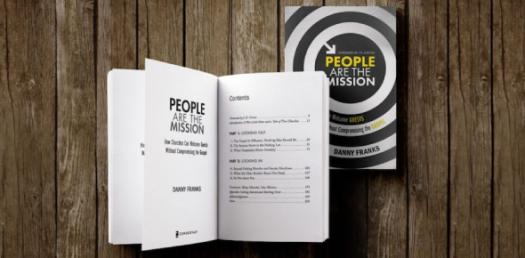# Book M Ch 3 Test

25 Questions | Total Attempts: 189Settings.

• 1.
Pressure is equal to
• A.

Force x area

• B.

Force / area

• C.

Mass / volume

• 2.
The SI unit of pressure is the
• A.

Millibar

• B.

Newton

• C.

Pascal

• 3.
If the area decreases, the pressure
• A.

Increases

• B.

Decreases

• C.

Remains the same

• 4.
A pascal is
• A.

1 M/n

• B.

1 N/m2

• C.

1 kg/m

• 5.
A tall thin glass and a wide short glass both have equal amounts of water. The pressure on the bottom of the tall thin glass is ___ than the pressure on the bottom of the wide short glass.
• A.

Greater than

• B.

Less than

• C.

The same as

• 6.
A substance that has no definate shape and has the ability to flow
• A.

Solid

• B.

Fluid

• C.

Gas

• 7.
An instrument used to measure air pressure
• A.

Barometer

• B.

Anemometer

• C.

• 8.
Place a check mark on all of the following that are fluids
• A.

Cloth

• B.

Water

• C.

Ice

• D.

Paper

• E.

Soup

• F.

Motor oil

• 9.
Place a check mark on all of the following that ARE NOT fluids
• A.

Helium

• B.

Paper clip

• C.

Plasma

• D.

Wet paint

• E.

Ice

• F.

Cloth

• 10.
The pressure exerted on an object by a fluid is always perpendicular to the surface of the object
• A.

True

• B.

False

• 11.
The bouyant force depends upon the shape of the object in the fluid.
• A.

True

• B.

False

• 12.
Density is force/unit area
• A.

True

• B.

False

• 13.
As you increase the area over which a force is applied, you decrease the pressure
• A.

True

• B.

False

• 14.
For an object to sink in a fluid there must be an unbalanced force
• A.

True

• B.

False

• 15.
Bouyant force acts in all directions
• A.

True

• B.

False

• 16.
Pascal's principle explains hydraulic systems
• A.

True

• B.

False

• 17.
The upward force on an airplane's wing is thrust
• A.

True

• B.

False

• 18.
The pressure of an object is equal to the ___ applied to the surface of the object.
• A.

Force per unit of volume

• B.

Force per unit of area

• C.

Mass per unit of volume

• D.

Mass per unit of area

• 19.
If you were walking in the snow and add snowshoes to your boots, the pressure on the snow would ___.
• A.

Become less

• B.

Become greater

• C.

Not change

• D.

Decrease with the depth of snow

• 20.
A fluid always
• A.

Has definate shape and volume

• B.

Can be compressed and flows

• C.

Has no definate shape and flows

• D.

Fills the volume of the container

• 21.
The bouyant force
• A.

Exerts a downward force on an object

• B.

Exerts an upwards force on an object

• C.

Is less than the density of water

• D.

Resists the motion of an object

• 22.
The bouyant force on an object is equal to the __.
• A.

Volume of the fluid it displaces

• B.

Weight of the fluid it displaces

• C.

Volume of the object

• D.

Weight of the object

• 23.
As the speed of a fluid decreases, the pressure it exerts ____.
• A.

Increases

• B.

Decreases

• C.

Remains the same

• D.

Becomes infinate

• 24.
If you squeeze the bottom of a closed tube of toothpaste, the pressure is increased ___.
• A.

On the sides of the tube

• B.

Everywhere in the tube

• C.

On the bottom of the tube

• D.

On the top of the tube

• 25.
In a hydraulic system, the pressure on the large piston is ___ the pressure on the small piston
• A.

Greater than

• B.

Less than

• C.

The same

• D.

Not related to

Related Topics